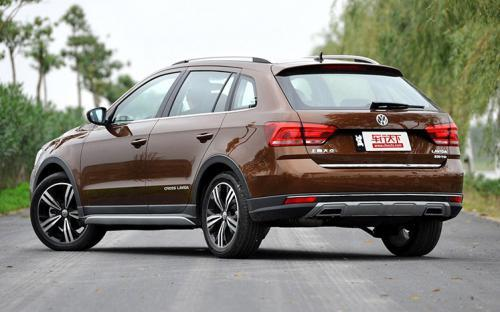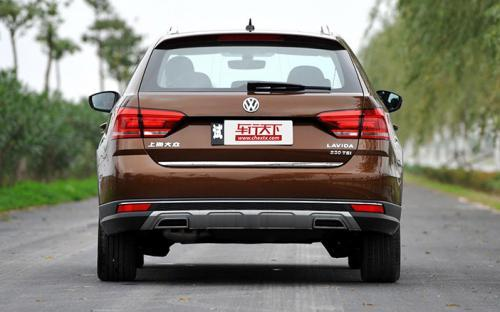## 上海大众 朗境0 种颜色可选2016款最低售价：14.89 万元起

4446(mm)1767(mm)1495(mm)##### 配置亮点：
• 胎压监测装置

• ISOFIX儿童座椅接口

• 车身稳定控制(ESC/ESP/DSC等)

• 电动天窗

• 定速巡航

• 后倒车雷达

• 真皮座椅

• GPS导航系统

• 氙气大灯

• 后视镜加热

2016款 230TSI DSG豪华版 (122张)上海大众 朗境 在售车型

排量 车型 厂商指导价 本地最低报价 购车工具
1.6L 1.6L 自动舒适型 6挡手自一体 14.89万 询底价+对比
1.6L 自动豪华型 6挡手自一体 16.19万 询底价+对比
1.4T 230TSI DSG舒适版 7挡双离合 15.89万 询底价+对比
230TSI DSG豪华版 7挡双离合 17.19万 询底价+对比

上海大众 朗境 经销商

查看更多 >>
更多 >>

本地降价排名

### 上海大众 朗境 动力加速

朗境 0-100公里加速时间分布在 9.3-12.3秒 属于 民用级

动力级别 加速时间 车型
民用级(2款) 12.3s 1.6L 自动舒适型1.6L 自动豪华型1.6L 自动型
9.3s 230TSI DSG舒适版230TSI DSG豪华版1.4TSI DSG

上海大众 朗境 视频

上海大众 朗境 新闻资讯

# 上海大众新款朗境上市 售14.89万元起

国产新车 超过9458次关注

在本届广州车展中，上海大众新款朗境车型正式上市，售价区间为14.89-17.19万元。朗境为朗逸家族的跨界车型，新车的变化并不明显。新车搭载的是1.6L以及1.4T这两款发...

# 车展体验上海大众朗境 预计将年内上市

导购 超过3643次关注

今年6月29日，上海大众朗行刚刚上市（售价：11.59-16.39万元），较大的后备厢让其看上去很像一辆旅行车，加上还算亲民的售价，受关注程度非常高。两个月后，朗行的C...

# 上海大众新车 朗境亮相广州车展

新闻 超过2244次关注

上海大众朗境已正式上市，售价为15.79-16.89万元。该车是基于朗行进行升级打造的。与朗行相比，朗境在外观方面增加了越野风格的套件，从而突出跨界风格。内饰方面，...

# 朗境领衔 上海大众四款新车将5日上市

新闻 超过2420次关注

上海大众将在广州举行lavidafamily的上市仪式，预计价格公布时间在20:00-21:30期间，届时将有朗境、朗逸蓝驱版、朗逸运动版以及朗行运动版四款车型公布价格并正式上市。

# 上海大众朗境上市 售15.79-16.89万元

新闻 超过2585次关注

上海大众举行了lavidafamily的上市仪式，期间朗境车型正式公布价格。此次上市的朗境共有2款车型，售价区间为15.79-16.89万元。

# 大众再推新口味 实拍上海大众朗境1.4T

车系文章 超过3239次关注

在上海大众朗逸+朗行两款车型加在一起的月销量突破了4万台大关，牢牢占据了紧凑型车销量霸主地位（福克斯合计销量30959台，位居第二），但上海大众似乎还不满足，又...

# cross风 行李架 试驾上海大众朗境

车系文章 超过4017次关注

Lavida(朗逸)的上市让几乎所有紧凑型车市场的对手都黯然失色，上市5年一百万辆的销售量也足够说明一切了。不过上海大众并不打算给对手任何喘息的机会，在今年连续推...

猜你喜欢

﻿
• 快速找车
• 选择品牌
• 选择品牌
• A  奥迪
• A  阿斯顿·马丁
• A  阿尔法·罗密欧
• B  宝沃
• B  布加迪
• B  巴博斯
• B  保时捷
• B  宾利
• B  奔驰
• B  宝马
• B  本田
• B  别克
• B  标致
• B  比亚迪
• B  宝骏
• B  北汽制造
• B  北汽新能源
• B  北汽幻速
• B  北汽威旺
• B  北京汽车
• B  奔腾
• B  北汽绅宝
• C  长安
• C  长安商用
• C  长城
• C  昌河
• D  大众
• D  道奇
• D  DS
• D  东南
• D  东风风神
• D  东风风行
• D  东风小康
• D  东风风度
• D  东风
• F  福特
• F  丰田
• F  菲亚特
• F  法拉利
• F  福田
• F  福迪
• F  福汽启腾
• G  观致
• G  广汽传祺
• G  广汽吉奥
• G  GMC
• H  红旗
• H  汉腾汽车
• H  哈弗
• H  哈飞
• H  海格
• H  海马
• H  华颂
• H  黄海
• H  华泰
• H  恒天
• J  吉利汽车
• J  捷豹
• J  Jeep
• J  江淮
• J  江铃
• J  金杯
• J  九龙
• J  金旅
• K  凯翼
• K  凯迪拉克
• K  克莱斯勒
• K  科尼塞克
• K  卡威
• K  开瑞
• L  路虎
• L  林肯
• L  劳斯莱斯
• L  兰博基尼
• L  雷克萨斯
• L  铃木
• L  雷诺
• L  理念
• L  力帆
• L  莲花汽车
• L  猎豹
• L  路特斯
• L  陆风
• M  马自达
• M  MG
• M  MINI
• M  玛莎拉蒂
• M  摩根
• M  迈凯轮
• N  纳智捷
• O  欧宝
• O  讴歌
• O  欧朗
• Q  奇瑞
• Q  起亚
• Q  启辰
• R  日产
• R  荣威
• R  瑞麒
• S  三菱
• S  斯威汽车
• S  萨博
• S  smart
• S  斯柯达
• S  斯巴鲁
• S  思铭
• S  双龙
• S  上汽大通
• S  双环
• T  特斯拉
• T  腾势
• W  沃尔沃
• W  五菱汽车
• W  五十铃
• W  威兹曼
• W  威麟
• X  现代
• X  雪佛兰
• X  雪铁龙
• X  西雅特
• Y  一汽
• Y  英菲尼迪
• Y  英致
• Y  依维柯
• Y  野马汽车
• Y  永源
• Z  众泰
• Z  中华
• Z  中兴
• Z  知豆
• 选择车系
• 选择车系
• 车型对比
• 选择品牌
• 选择品牌
• A  奥迪
• A  阿斯顿·马丁
• A  阿尔法·罗密欧
• B  宝沃
• B  布加迪
• B  巴博斯
• B  保时捷
• B  宾利
• B  奔驰
• B  宝马
• B  本田
• B  别克
• B  标致
• B  比亚迪
• B  宝骏
• B  北汽制造
• B  北汽新能源
• B  北汽幻速
• B  北汽威旺
• B  北京汽车
• B  奔腾
• B  北汽绅宝
• C  长安
• C  长安商用
• C  长城
• C  昌河
• D  大众
• D  道奇
• D  DS
• D  东南
• D  东风风神
• D  东风风行
• D  东风小康
• D  东风风度
• D  东风
• F  福特
• F  丰田
• F  菲亚特
• F  法拉利
• F  福田
• F  福迪
• F  福汽启腾
• G  观致
• G  广汽传祺
• G  广汽吉奥
• G  GMC
• H  红旗
• H  汉腾汽车
• H  哈弗
• H  哈飞
• H  海格
• H  海马
• H  华颂
• H  黄海
• H  华泰
• H  恒天
• J  吉利汽车
• J  捷豹
• J  Jeep
• J  江淮
• J  江铃
• J  金杯
• J  九龙
• J  金旅
• K  凯翼
• K  凯迪拉克
• K  克莱斯勒
• K  科尼塞克
• K  卡威
• K  开瑞
• L  路虎
• L  林肯
• L  劳斯莱斯
• L  兰博基尼
• L  雷克萨斯
• L  铃木
• L  雷诺
• L  理念
• L  力帆
• L  莲花汽车
• L  猎豹
• L  路特斯
• L  陆风
• M  马自达
• M  MG
• M  MINI
• M  玛莎拉蒂
• M  摩根
• M  迈凯轮
• N  纳智捷
• O  欧宝
• O  讴歌
• O  欧朗
• Q  奇瑞
• Q  起亚
• Q  启辰
• R  日产
• R  荣威
• R  瑞麒
• S  三菱
• S  斯威汽车
• S  萨博
• S  smart
• S  斯柯达
• S  斯巴鲁
• S  思铭
• S  双龙
• S  上汽大通
• S  双环
• T  特斯拉
• T  腾势
• W  沃尔沃
• W  五菱汽车
• W  五十铃
• W  威兹曼
• W  威麟
• X  现代
• X  雪佛兰
• X  雪铁龙
• X  西雅特
• Y  一汽
• Y  英菲尼迪
• Y  英致
• Y  依维柯
• Y  野马汽车
• Y  永源
• Z  众泰
• Z  中华
• Z  中兴
• Z  知豆
• 选择车系
• 选择车系
• 选择车型
• 选择车型
• 意见反馈